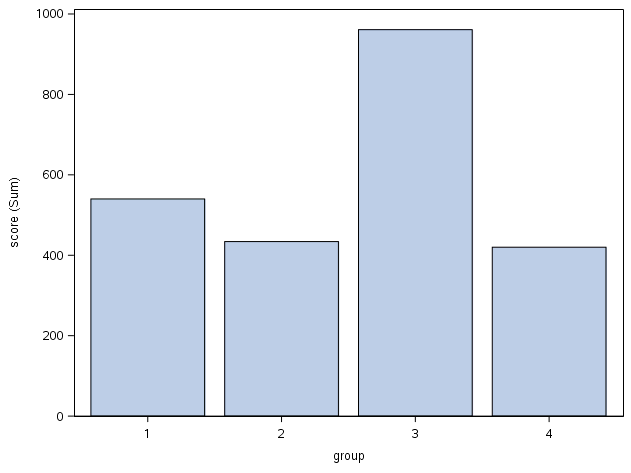## overlay histograms only for some group members

Hi All,

I have a data set as below: I want to compare the histograms of the scores of two different groups with all possible combinations. For example,

comparison of  scores for groups ts=A and tc=B;

comparison of  scores for groups ts=B and tc=B;

comparison of  scores for  groups ts=B and tc=A;

comparison of  scores for groups ts=A and tc=A;

So, there will be 4 different overlaying histograms. Could you help to draw overlaying histograms of these groups' scores?

Thank you,

score ts tc matching
180 A B 0
200 B B 1
360 A B 0
420 A A 1
500 B A 0
234 B B 1
461 B A 0

2 REPLIES 2

## Re: overlay histograms only for some group members

``````data have;
input score ts\$ tc\$ matching;
cards;
180 A B 0
200 B B 1
360 A B 0
420 A A 1
500 B A 0
234 B B 1
461 B A 0
;

data want;
set have;
if ts='A' and tc='B' then group='1';
if ts='B' and tc='B' then group='2';
if ts='B' and tc='A' then group='3';
if ts='A' and tc='A' then group='4';
run;

proc sgplot data=want;
vbar group/response=score;
run;``````Thanks,
Jag

## Re: overlay histograms only for some group members

I don't think the problem is formulated correctly. To get two overlayed histograms, you need some observations that belong to one group and other (disjoint) observations in another group. But in your example,  ts='A' includes observations for both tc='A' and tc='B'. So you can't overlay the histograms. There are not four histograms to overlay.

Two things that you can do:

1. create two overlapping histograms. The first compares tc='A' and tc='B' given that ts='A'. The second compares tc='A' and tc='B' given that ts='B'.

2. create a panel of histograms of the four groups, which are the joint levels of (ts, tc).

Both of these options are shown below on some simulated data:

``````data Have;
do ts = 'A', 'B';
do tc = 'A', 'B';
do k=1 to 50;
score = 3*(ts='A') + -1*(tc='A') + rand("Normal");
output;
end;
end;
end;
run;

/* 1. Given a value for ts, overlay the distributions for levels of tc */
proc sgplot data=Have;
by ts;
histogram score / group=tc transparency=0.5;
run;

/* 2. Panel the four distributions */
data Want;
set Have;
group = cats("ts=", ts, "; tc=", tc);
run;

proc sgpanel data=Want;
panelby group / onepanel columns=1 novarname;
histogram score;
run;``````
Discussion stats
• 2 replies
• 330 views
• 0 likes
• 3 in conversation